# Selina Solutions Concise Maths Class 7 Chapter 7: Unitary Method (Including Time and Work) Exercise 7C

Selina Solutions Concise Maths Class 7 Chapter 7 Unitary Method (Including Time and Work) Exercise 7C has problems on finding the time and work in the given set of questions. Students cannot mug up the concepts explained in the textbook. Regular practise is the major key in obtaining good marks in the annual exam. The solutions are prepared by subject matter experts, keeping in mind the understanding capacity of students. In order to score well in the exam, students can use Selina Solutions Concise Maths Class 7 Chapter 7 Unitary Method (Including Time and Work) Exercise 7C free PDF, from the links given here.

## Selina Solutions Concise Maths Class 7 Chapter 7: Unitary Method (Time and Work) Exercise 7C Download PDF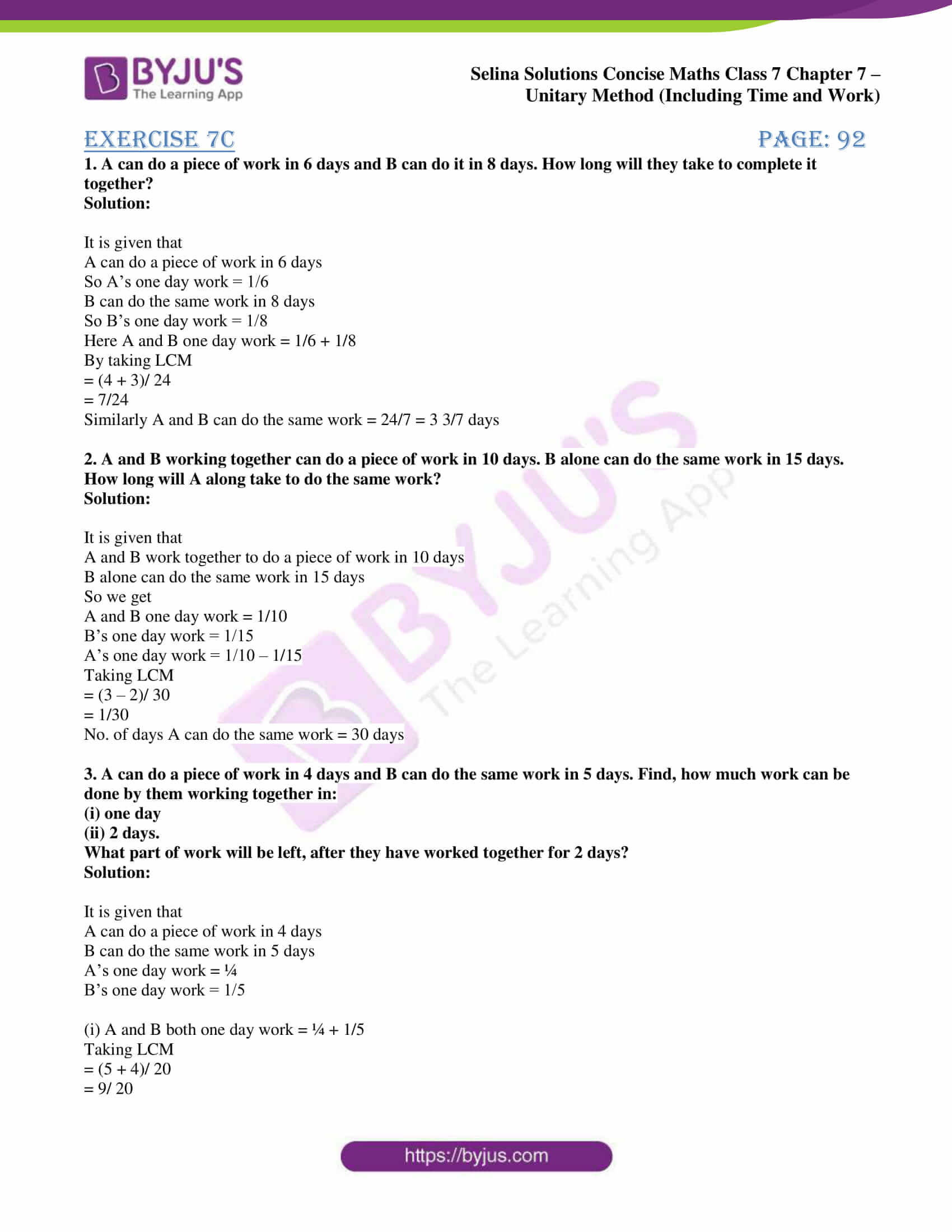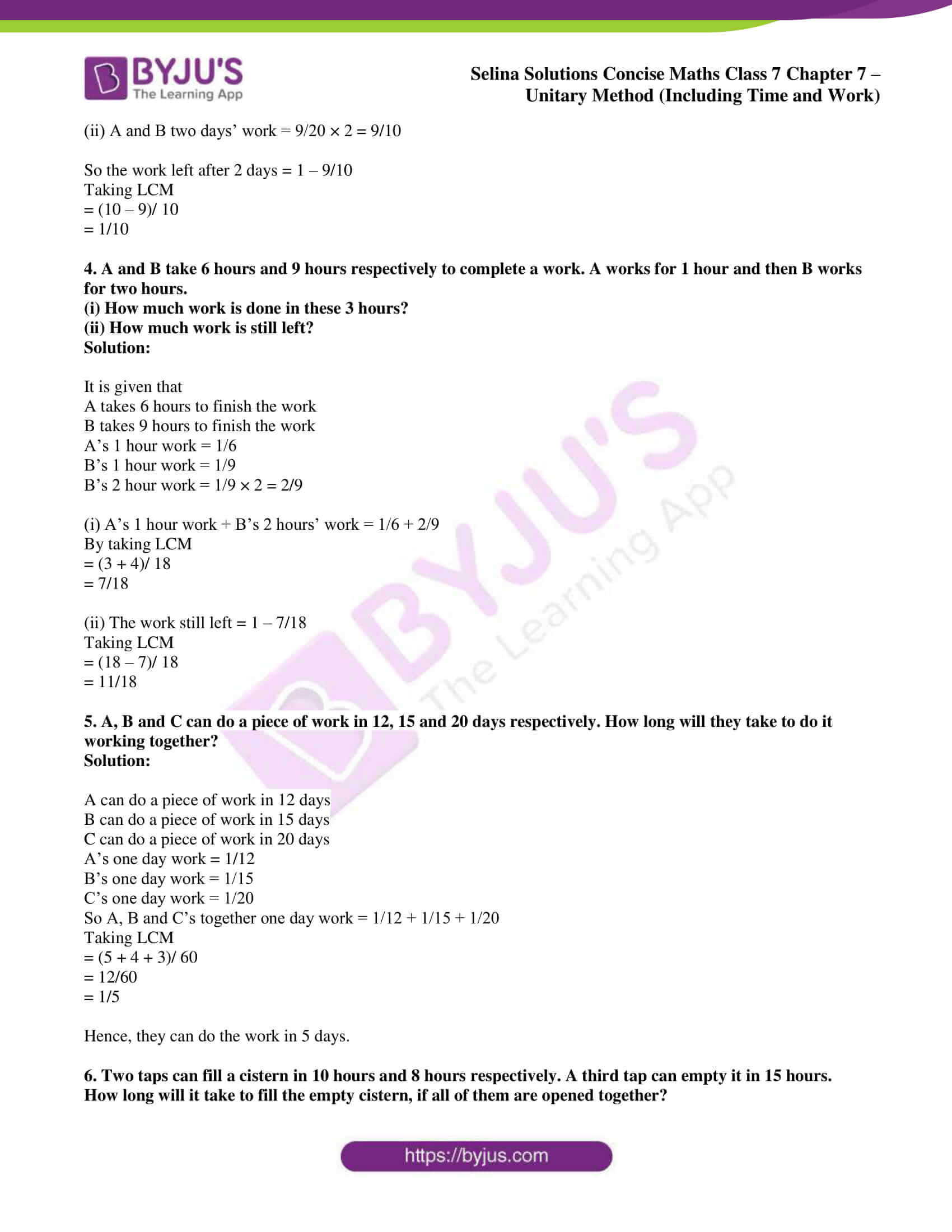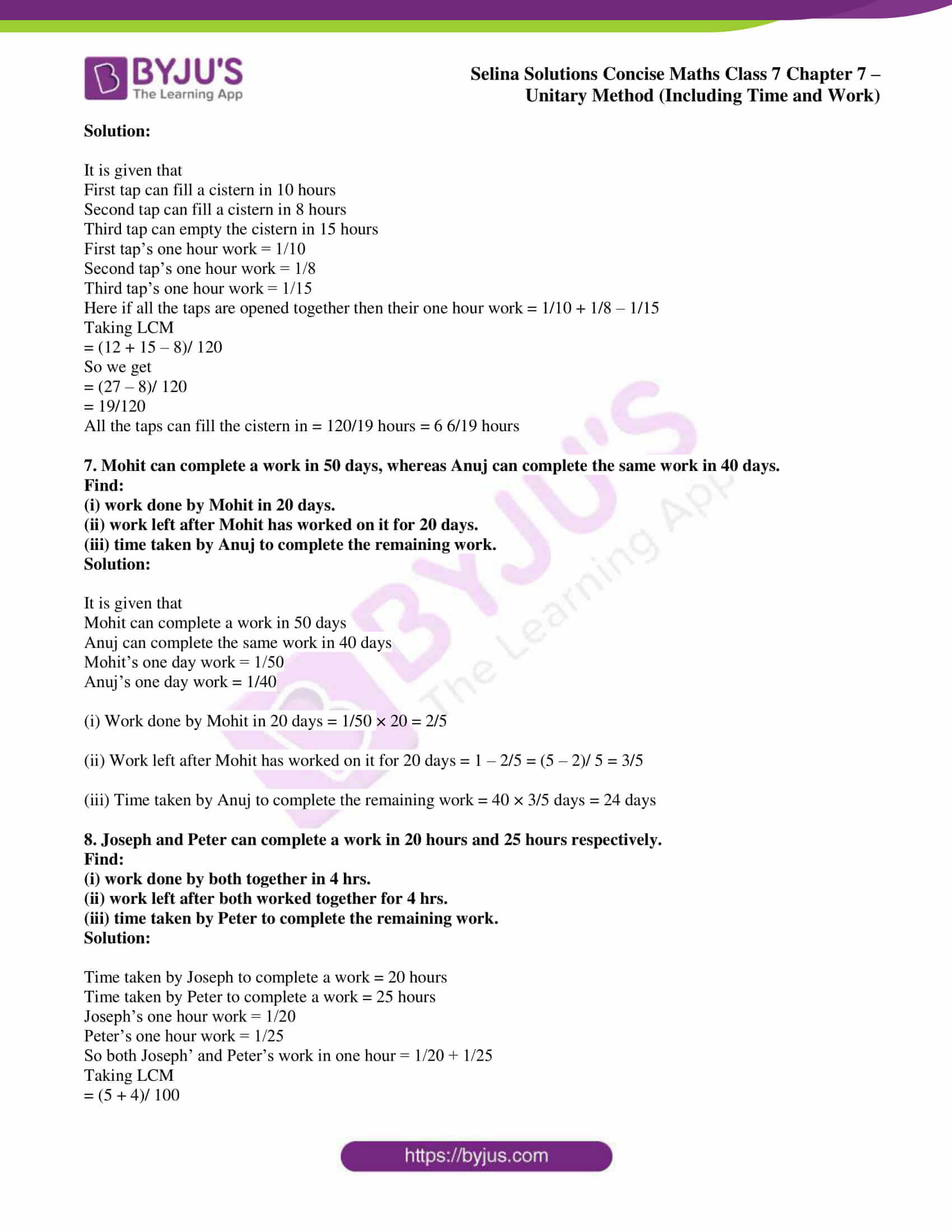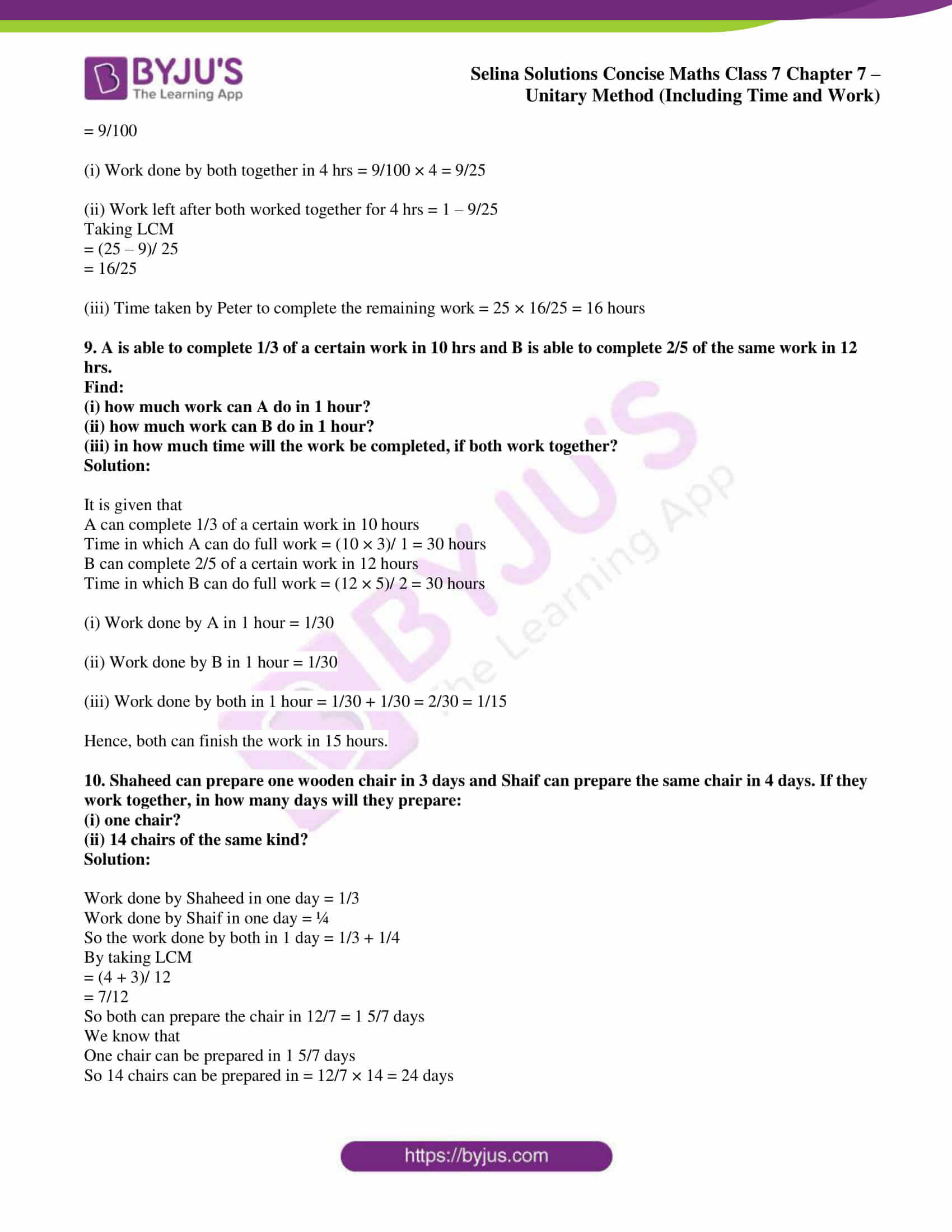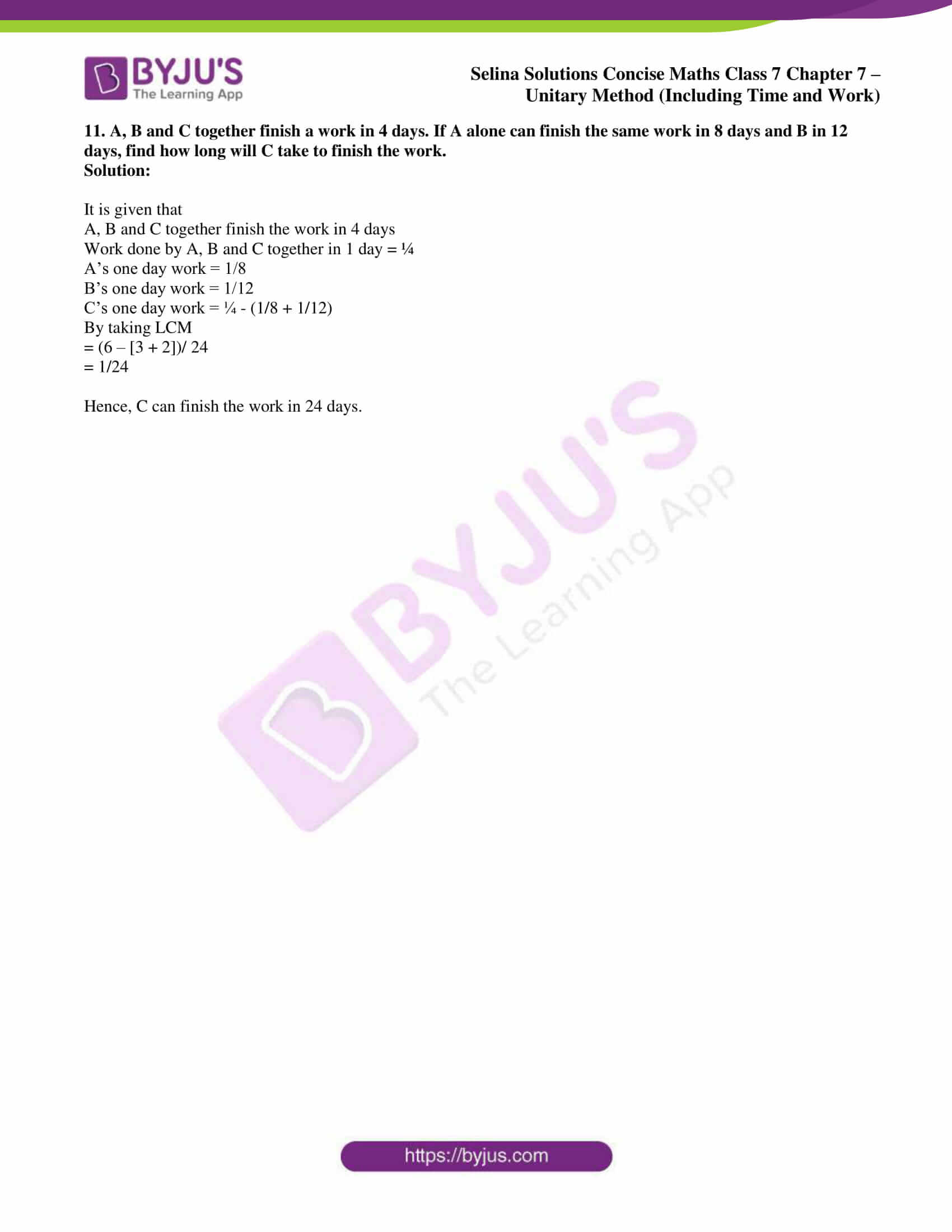### Access Selina Solutions Concise Maths Class 7 Chapter 7: Unitary Method (Time and Work) Exercise 7C

1. A can do a piece of work in 6 days and B can do it in 8 days. How long will they take to complete it together?

Solution:

It is given that

A can do a piece of work in 6 days

So A’s one day work = 1/6

B can do the same work in 8 days

So B’s one day work = 1/8

Here A and B one day work = 1/6 + 1/8

By taking LCM

= (4 + 3)/ 24

= 7/24

Similarly A and B can do the same work = 24/7 = 3 3/7 days

2. A and B working together can do a piece of work in 10 days. B alone can do the same work in 15 days. How long will A along take to do the same work?

Solution:

It is given that

A and B work together to do a piece of work in 10 days

B alone can do the same work in 15 days

So we get

A and B one day work = 1/10

B’s one day work = 1/15

A’s one day work = 1/10 – 1/15

Taking LCM

= (3 – 2)/ 30

= 1/30

No. of days A can do the same work = 30 days

3. A can do a piece of work in 4 days and B can do the same work in 5 days. Find, how much work can be done by them working together in:

(i) one day

(ii) 2 days.

What part of work will be left, after they have worked together for 2 days?

Solution:

It is given that

A can do a piece of work in 4 days

B can do the same work in 5 days

A’s one day work = ¼

B’s one day work = 1/5

(i) A and B both one day work = ¼ + 1/5

Taking LCM

= (5 + 4)/ 20

= 9/ 20

(ii) A and B two days’ work = 9/20 × 2 = 9/10

So the work left after 2 days = 1 – 9/10

Taking LCM

= (10 – 9)/ 10

= 1/10

4. A and B take 6 hours and 9 hours respectively to complete a work. A works for 1 hour and then B works for two hours.

(i) How much work is done in these 3 hours?

(ii) How much work is still left?

Solution:

It is given that

A takes 6 hours to finish the work

B takes 9 hours to finish the work

A’s 1 hour work = 1/6

B’s 1 hour work = 1/9

B’s 2 hour work = 1/9 × 2 = 2/9

(i) A’s 1 hour work + B’s 2 hours’ work = 1/6 + 2/9

By taking LCM

= (3 + 4)/ 18

= 7/18

(ii) The work still left = 1 – 7/18

Taking LCM

= (18 – 7)/ 18

= 11/18

5. A, B and C can do a piece of work in 12, 15 and 20 days respectively. How long will they take to do it working together?

Solution:

A can do a piece of work in 12 days

B can do a piece of work in 15 days

C can do a piece of work in 20 days

A’s one day work = 1/12

B’s one day work = 1/15

C’s one day work = 1/20

So A, B and C’s together one day work = 1/12 + 1/15 + 1/20

Taking LCM

= (5 + 4 + 3)/ 60

= 12/60

= 1/5

Hence, they can do the work in 5 days.

6. Two taps can fill a cistern in 10 hours and 8 hours respectively. A third tap can empty it in 15 hours. How long will it take to fill the empty cistern, if all of them are opened together?

Solution:

It is given that

First tap can fill a cistern in 10 hours

Second tap can fill a cistern in 8 hours

Third tap can empty the cistern in 15 hours

First tap’s one hour work = 1/10

Second tap’s one hour work = 1/8

Third tap’s one hour work = 1/15

Here if all the taps are opened together then their one hour work = 1/10 + 1/8 – 1/15

Taking LCM

= (12 + 15 – 8)/ 120

So we get

= (27 – 8)/ 120

= 19/120

All the taps can fill the cistern in = 120/19 hours = 6 6/19 hours

7. Mohit can complete a work in 50 days, whereas Anuj can complete the same work in 40 days.

Find:

(i) work done by Mohit in 20 days.

(ii) work left after Mohit has worked on it for 20 days.

(iii) time taken by Anuj to complete the remaining work.

Solution:

It is given that

Mohit can complete a work in 50 days

Anuj can complete the same work in 40 days

Mohit’s one day work = 1/50

Anuj’s one day work = 1/40

(i) Work done by Mohit in 20 days = 1/50 × 20 = 2/5

(ii) Work left after Mohit has worked on it for 20 days = 1 – 2/5 = (5 – 2)/ 5 = 3/5

(iii) Time taken by Anuj to complete the remaining work = 40 × 3/5 days = 24 days

8. Joseph and Peter can complete a work in 20 hours and 25 hours respectively.

Find:

(i) work done by both together in 4 hrs.

(ii) work left after both worked together for 4 hrs.

(iii) time taken by Peter to complete the remaining work.

Solution:

Time taken by Joseph to complete a work = 20 hours

Time taken by Peter to complete a work = 25 hours

Joseph’s one hour work = 1/20

Peter’s one hour work = 1/25

So both Joseph’ and Peter’s work in one hour = 1/20 + 1/25

Taking LCM

= (5 + 4)/ 100

= 9/100

(i) Work done by both together in 4 hrs = 9/100 × 4 = 9/25

(ii) Work left after both worked together for 4 hrs = 1 – 9/25

Taking LCM

= (25 – 9)/ 25

= 16/25

(iii) Time taken by Peter to complete the remaining work = 25 × 16/25 = 16 hours

9. A is able to complete 1/3 of a certain work in 10 hrs and B is able to complete 2/5 of the same work in 12 hrs.

Find:

(i) how much work can A do in 1 hour?

(ii) how much work can B do in 1 hour?

(iii) in how much time will the work be completed, if both work together?

Solution:

It is given that

A can complete 1/3 of a certain work in 10 hours

Time in which A can do full work = (10 × 3)/ 1 = 30 hours

B can complete 2/5 of a certain work in 12 hours

Time in which B can do full work = (12 × 5)/ 2 = 30 hours

(i) Work done by A in 1 hour = 1/30

(ii) Work done by B in 1 hour = 1/30

(iii) Work done by both in 1 hour = 1/30 + 1/30 = 2/30 = 1/15

Hence, both can finish the work in 15 hours.

10. Shaheed can prepare one wooden chair in 3 days and Shaif can prepare the same chair in 4 days. If they work together, in how many days will they prepare:

(i) one chair?

(ii) 14 chairs of the same kind?

Solution:

Work done by Shaheed in one day = 1/3

Work done by Shaif in one day = ¼

So the work done by both in 1 day = 1/3 + 1/4

By taking LCM

= (4 + 3)/ 12

= 7/12

So both can prepare the chair in 12/7 = 1 5/7 days

We know that

One chair can be prepared in 1 5/7 days

So 14 chairs can be prepared in = 12/7 × 14 = 24 days

11. A, B and C together finish a work in 4 days. If A alone can finish the same work in 8 days and B in 12 days, find how long will C take to finish the work.

Solution:

It is given that

A, B and C together finish the work in 4 days

Work done by A, B and C together in 1 day = ¼

A’s one day work = 1/8

B’s one day work = 1/12

C’s one day work = ¼ – (1/8 + 1/12)

By taking LCM

= (6 – [3 + 2])/ 24

= 1/24

Hence, C can finish the work in 24 days.